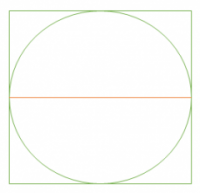GMAT Question of the Day: Daily via email | Daily via Instagram New to GMAT Club? Watch this Video

 It is currently 05 Aug 2020, 18:06### GMAT Club Daily Prep

#### Thank you for using the timer - this advanced tool can estimate your performance and suggest more practice questions. We have subscribed you to Daily Prep Questions via email.

Customized
for You

we will pick new questions that match your level based on your Timer History

Track

every week, we’ll send you an estimated GMAT score based on your performance

Practice
Pays

we will pick new questions that match your level based on your Timer History

#### Not interested in getting valuable practice questions and articles delivered to your email? No problem, unsubscribe here.# If the circumference of a circle inscribed in a square is 25π, what is

Author Message
TAGS:

### Hide Tags

Math ExpertV
Joined: 02 Sep 2009
Posts: 65808
If the circumference of a circle inscribed in a square is 25π, what is  [#permalink]

### Show Tags

1
1900:00

Difficulty:5% (low)

Question Stats:81% (00:56) correct19% (00:58) wrongbased on 1119 sessions

### HideShow timer Statistics

If the circumference of a circle inscribed in a square is 25π, what is the perimeter of the square?

A. 20

B. 25

C. 40

D. 50

E. 100

NEW question from GMAT® Official Guide 2019

(PS12542)

_________________
InternB
Joined: 21 May 2017
Posts: 38
Re: If the circumference of a circle inscribed in a square is 25π, what is  [#permalink]

### Show Tags

3

Diameter of circle = Side of square.
Let diameter be d and side be s.
Circumference = πd = 25π
So, d = 25 = s.
Perimeter = 4s = 100

Sent from my ONEPLUS A3003 using GMAT Club Forum mobile app
Current StudentB
Joined: 03 Aug 2014
Posts: 18
Schools: Kellogg '21 (A)
Re: If the circumference of a circle inscribed in a square is 25π, what is  [#permalink]

### Show Tags

2
πD = 25π => diameter is 25.
Therefore, side of square = 25.
Perimeter = 4*side = 100

Sent from my Pixel using GMAT Club Forum mobile app
e-GMAT RepresentativeV
Joined: 04 Jan 2015
Posts: 3440
Re: If the circumference of a circle inscribed in a square is 25π, what is  [#permalink]

### Show Tags

Solution

Given:
• The circumference of a circle inscribed in a square is 25π

To find:
• The perimeter of the square

Approach and Working:
When a circle is inscribed in a square, the diameter of the circle is equal to the length of the side of the squareIf r is the radius of the circle, then
• 2πr = 25π
Or, 2r = 25

Therefore, we can say, length of the side of the square = 25
• Perimeter of the square = 25 * 4 = 100

Hence, the correct answer is option E.

_________________
GMAT Club LegendV
Joined: 11 Sep 2015
Posts: 4999
GMAT 1: 770 Q49 V46
Re: If the circumference of a circle inscribed in a square is 25π, what is  [#permalink]

### Show Tags

Top Contributor
Bunuel wrote:
If the circumference of a circle inscribed in a square is 25π, what is the perimeter of the square?

A. 20

B. 25

C. 40

D. 50

E. 100

We have the following:The perimeter of the circle = 25π

We can see that the perimeter of the square is GREATER THAN the perimeter of the circle

25π ≈ (25)(3) ≈ 75
So, the perimeter of the square is GREATER THAN 25π

Cheers,
Brent
_________________
If you enjoy my solutions, you'll love my GMAT prep course.Target Test Prep RepresentativeG
Affiliations: Target Test Prep
Joined: 04 Mar 2011
Posts: 2800
Re: If the circumference of a circle inscribed in a square is 25π, what is  [#permalink]

### Show Tags

Bunuel wrote:
If the circumference of a circle inscribed in a square is 25π, what is the perimeter of the square?

A. 20

B. 25

C. 40

D. 50

E. 100

When a circle is inscribed in a square, the diameter of the circle is equal to the side of the square.

Since circumference = πd, we have:

πd = 25π

d = 25 = side of the square

Thus, the perimeter of the square is 25 x 4 = 100.

_________________

# Jeffrey Miller | Head of GMAT Instruction | Jeff@TargetTestPrep.com

250 REVIEWS

5-STAR RATED ONLINE GMAT QUANT SELF STUDY COURSE

NOW WITH GMAT VERBAL (BETA)

See why Target Test Prep is the top rated GMAT quant course on GMAT Club. Read Our Reviews

InternB
Joined: 01 Mar 2019
Posts: 34
Location: United States
Schools: Owen '22 (M\$)
If the circumference of a circle inscribed in a square is 25π, what is  [#permalink]

### Show Tags

 Find Diameter

Since the diameter of a circle is equal to the side of a square, finding the circle's diameter will allow us to calculate the square's perimeter.

$$Circumference = Diameter \times \pi$$

$$\frac{25\pi}{\pi} = Diameter$$

$$Diameter = 25$$

 Calculate Perimeter

$$Perimeter = 4 \times Side$$

$$Side \equiv Diameter$$

$$25\times 4 = 100$$

ManagerB
Joined: 24 Sep 2018
Posts: 131
Location: India
Concentration: Entrepreneurship, Marketing
Re: If the circumference of a circle inscribed in a square is 25π, what is  [#permalink]

### Show Tags

The perimeter of the circle = 25π

2πr = 25π
So r= 25/2 so Diameter = 2* 25/2 = 25

So the perimeter of the square = 4a = 4* 25 = 100Re: If the circumference of a circle inscribed in a square is 25π, what is   [#permalink] 17 Jan 2020, 20:15

# If the circumference of a circle inscribed in a square is 25π, what is